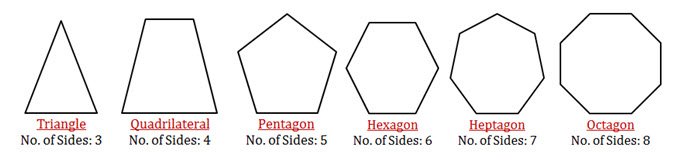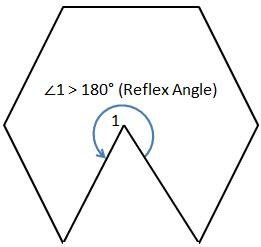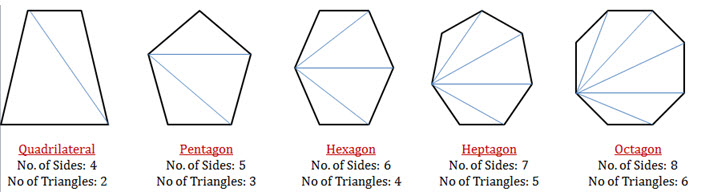In elementary geometry, a polygon is a plane figure that is bounded by a finite chain of straight line segments closing in a loop to form a closed chain or circuit. Or simply a closed plane figure bounded by three or more line segment to form a closed loop is called a polygon.Nomenclature:

• The line segments forming the polygon are called sides.
• The point of intersection of two line segments is called a vertex.
• Number of vertices of a polygon is equal to the number of line segments or sides.

Different types of Polygons:  This is based on the number of sides that the polygon has. Here are few examples:Diagonal of a Polygon: A line segment joining any two non-consecutive vertices is called

a diagonal of the polygon.

The dotted lines are diagonals of the shown polygons.

Interior and Exterior Angles of a Polygon: This is an important concept.$\angle 1 \ and\ \angle 2$ are interior angles. These are made by the two sides of the polygon.$\angle 3$ is called an exterior angle. This is formed by extending a side of the polygon as shown in the adjacent figure.

Just by looking at the figure you can tell that$\angle 2 + \angle 3 = 180^{\circ}$

Hence we can say that:

Exterior Angle + Adjacent Interior Angle$= 180^{\circ}$

Convex Polygon vs Concave PolygonIf the interior angle of the polygon is less than$= 180^{\circ}$, then it is called convex polygon. If you look at any of the polygons shown above, you will see that all the interior angles are less than$= 180^{\circ}$.

But there can be cases where the interior angle of a polygon could be more than$= 180^{\circ}$. Take a look at the adjacent figure. Here you will see that$\angle 1 > 180^{\circ}$ (which is a reflex angle).

Regular Polygon: A polygon that satisfies the following condition is called a regular polygon.

1. All sides are equal
2. All interior angles are equal
3. All exterior angles are equal

For a regular polygon with$n$ sides we have the following:$\displaystyle \text{Each Interior Angle }= \Bigg[\frac{(2n-4)\times 90^{\circ}}{n} \Bigg]^{\circ}$

Proof:

A polygon can be divided into$(n-2)$ triangles.

See a few examples in the adjacent figure.We know that the sum of the angles of a triangle is$= 180^{\circ}$.$\text{Therefore the sum of the interior angles }= (n-2) \times 180 = (2n - 4) \times 90^{\circ}$$\Rightarrow \displaystyle \text{Interior Angle } = \frac{(2n-4)\times 90^{\circ}}{n}$$\displaystyle \text{Each Exterior Angle } = \Big[\frac{360}{n} \Big]^{\circ}$

Proof:$\text{Sum of all Interior Angles + Sum of all Exterior Angles } = n \times 180 = n \times (2 \times 90)^{\circ}$$\text{Sum of all Exterior Angles }= n \times (2 \times 90)^{\circ} - (2n - 4) \times 90^{\circ}=360^{\circ}$$\displaystyle \text{Each Exterior Angle } = \Big[\frac{360}{n} \Big]^{\circ}$

From the above point 2, we can also say that$\displaystyle n = \Big[\frac{360}{\text{Each Exterior Angle}} \Big]^{\circ}$$\text{Exterior Angle + Adjacent Interior Angle }= 180^{\circ}$$\Rightarrow \text{Exterior Angle } =180^{\circ} - \text{Adjacent Interior Angle}$